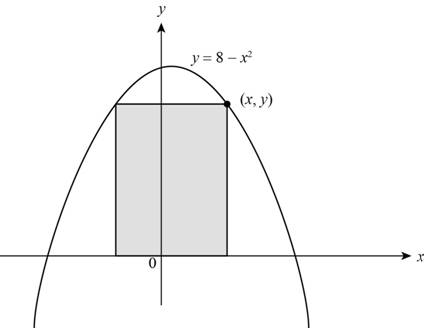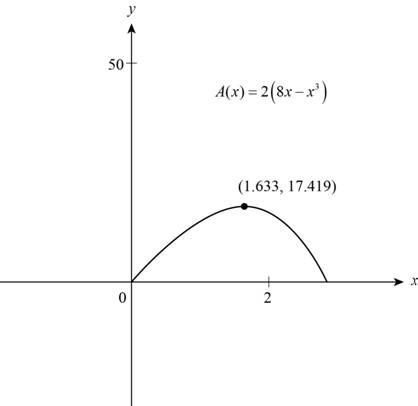# The dimension of a rectangle for which its area is maximum and two of its vertices are on x -axis and two vertices are on y = 8 − x 2### Precalculus: Mathematics for Calcu...

6th Edition
Stewart + 5 others
Publisher: Cengage Learning
ISBN: 9780840068071### Precalculus: Mathematics for Calcu...

6th Edition
Stewart + 5 others
Publisher: Cengage Learning
ISBN: 9780840068071

#### Solutions

Chapter 2, Problem 28P
To determine

## To find: The dimension of a rectangle for which its area is maximum and two of its vertices are on x-axis and two vertices are on y=8−x2

Expert Solution

The length of inscribed rectangle is 3.26 and height is 5.35 .

### Explanation of Solution

Given:

The given figure is,Figure (1)

Calculation:

Area of rectangle is,

A=(length)(width)=(l)(w)

From Figure (1) length is 2x and width is h .

Substitute 2x for l and y for w in equation (1)

A=(2x)(y)=2xy

Vertices of the rectangle are on y=8x2 .

Summarize the information in a table as shown below.

 In Words In Algebra Area l⋅w Length of side rectangle. 2x Height of the rectangle 8−x2

Use the information in the table and model the area in terms of x .

A=(l)(w)A(x)=(2x)(8x2)[y=8x2]=16x2x3=2(8xx3)

The function that models the area of given rectangle is,

A(x)=2(8xx3)

Sketch the graph of A(x) in first quadrant (because area and dimensions of rectangle is non-negative) using graphing calculator as shown below.Figure (2)

Observe from Figure (2) that function attains a maximum value when x is 1.63.

Substitute 1.63 for x in y=8x2 and solve for y.

y=8(1.63)2=82.65=5.35

The length of rectangle for maximum area is,

l=2x

Substitute 1.63 for x in above equation

l=3.26

Thus, the length of inscribed rectangle is 3.26 and height is 5.35 .

### Have a homework question?

Subscribe to bartleby learn! Ask subject matter experts 30 homework questions each month. Plus, you’ll have access to millions of step-by-step textbook answers!